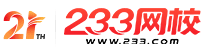#计算机二级

•扫描二维码进入

• 题 库扫描二维码进入

• 移动233网校移动

• 移动APP扫描二维码下载APP

## 2019年3月计算机二级C语言考试选择题巩固练习001

(1)下列数据结构中，属于非线性结构的是( )。

A.循环队列

B.带链队列

C.二叉树

D.带链栈

(2)下列数据结构中，能够按照“先进后出”原则存取数据的是( )。

A.循环队列

B.栈

C.队列

D.二叉树

(3)对于循环队列，下列叙述中正确的是( )。

A.队头指针是固定不变的

B.队头指针一定大于队尾指针

C.队头指针一定小于队尾指针

D.队头指针可以大于队尾指针，也可以小于队尾指针

(4)算法的空间复杂度是指( )。

A.算法在执行过程中所需要的计算机存储空间

B.算法所处理的数据量

C.算法程序中的语句或指令条数

D.算法在执行过程中所需要的临时工作单元数

(5)软件设计中划分模块的一个准则是( )。

A.低内聚低耦合

B.高内聚低耦合

C.低内聚高耦合

D.高内聚高耦台

(6)下列选项中不属于结构化程序设计原则的是( )。

A.可封装

B.自顶向下

C.模块化

D.逐步求精

(7)设有以下语句：

int a=1，b=2，c;c=a^(b<<2);执行后，c的值为( )。

A.7

B.9

C.8

D.6

(8)设有定义：

int a=1，b=2，C=3;

A)if(a>B.C=a，a=b，b=C;

B)B.if(a>B.{C=a，a=b，b=C;}

C)C.if(a>B.C=a;a=b;b=c;

D)D.if(a>B.{C=a;a=b;b=c;}

(9)在E―R图中，用来表示实体联系的图形是( )。

A.椭圆形

B.矩形

C.菱形

D.三角形

(10)以下关于宏的叙述中正确的是( )。

A.宏替换没有数据类型限制

B.宏定义必须位于源程序中所有语句之前

C.宏名必须用大写字母表示

D.宏调用比函数调用耗费时间

• 海量免费模拟试题，在线测试。

• 各科每年真题试卷，实战演练！

• 逐章逐节针对练习，专项突破。

• 每天10道最新试题，随机练习。

• QQ群号：806931213

扫描二维码加群• ##### 微信订阅号：kszx233

扫描二维码加群